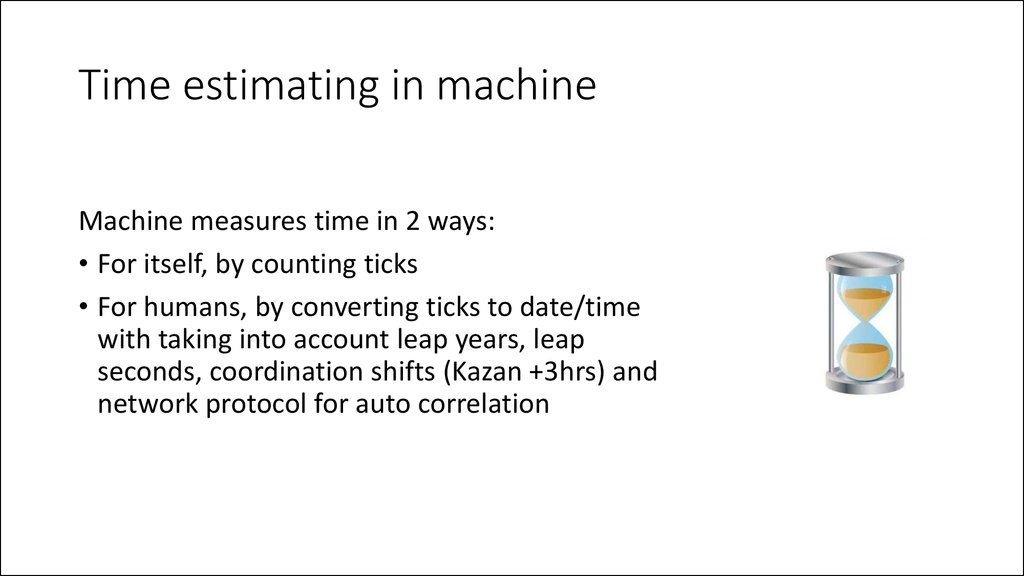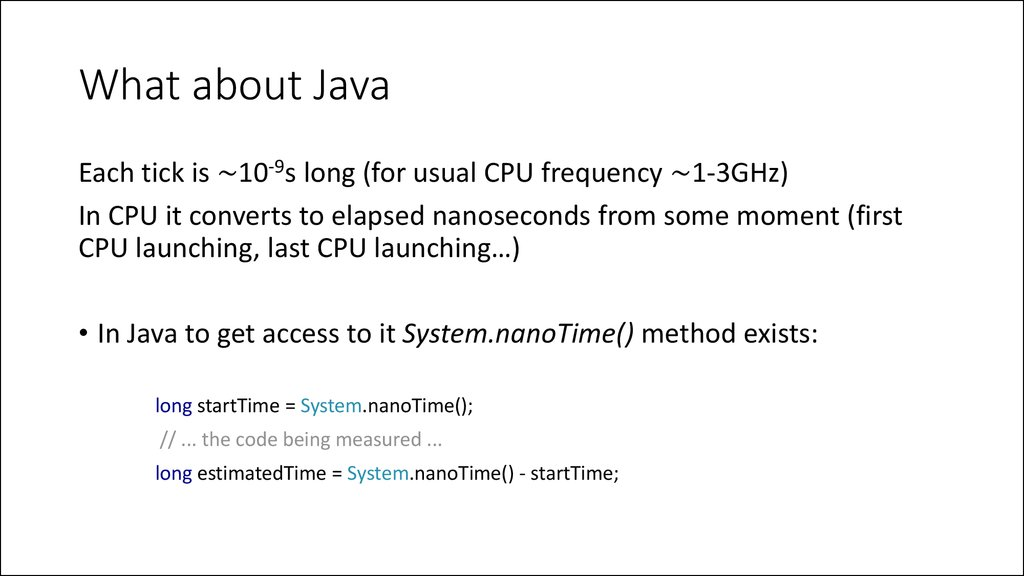24, August 2015
Kamil Salakhiev

## 2. Time estimating in machine

Machine measures time in 2 ways:
• For itself, by counting ticks
• For humans, by converting ticks to date/time
with taking into account leap years, leap
seconds, coordination shifts (Kazan +3hrs) and
network protocol for auto correlation

Each tick is ~10-9s long (for usual CPU frequency ~1-3GHz)
In CPU it converts to elapsed nanoseconds from some moment (first
CPU launching, last CPU launching…)
long startTime = System.nanoTime();
// ... the code being measured ...
long estimatedTime = System.nanoTime() - startTime;

## 4. Another method

Another way to calculate elapsed time is System.currentTimeMillis()
method:
long startTime = System.currentTimeMillis();
// ... do something ...
long estimatedTime = System.currentTimeMillis() – startTime;
Why long?

## 5. Storage estimating

• Storage refers to the data storage consumed in performing a given
task, whether primary (e.g., in RAM) or secondary (e.g., on a hard disk
drive)
• In Java to estimate consumed memory there is a
Runtime.getRuntime().totalMemory() method, that returns
the total amount of memory currently occupied for current objects
measured in bytes:
long start = Runtime.getRuntime().totalMemory();
System.out.println("start = " + start); // prints 64487424
int arr[] = new int;
long finish = Runtime.getRuntime().totalMemory();
System.out.println("finish = " + finish); // prints 464519168

## 6. The RAM model of computation

The RAM model of computation estimate algorithm according the following
rules:
• Each simple operation (+, *, –, =, if, call) takes exactly one time step.
• Loops and procedures are not considered as simple operations.
• Each memory access takes exactly one time step
Example:
for (int i = 0; i < n; i++) {
x++;
}
Takes n steps

## 7. Big O notation

• In Big O notation we are interested in the determining the order of
magnitude of time complexity of an algorithm

## 8. Calculate n-th Fibonacci number (n = 0)

Number of steps: 5

## 9. Calculate n-th Fibonacci number (n = 1)

Number of steps: 6

## 10. Calculate n-th Fibonacci number (n > 1)

Calculate n-th Fibonacci number (n > 1)
Number of steps: 9 + n + 3(n-1) = 4n + 6

## 11. Fibonacci number

• For n = 0 Number of steps: 5
• For n = 1 Number of steps: 6
• For n > 1 Number of steps: 4n – 6
In Big O notation we take the highest complexity in terms of order,
remove constants and variables with order lower than the highest one.
Thus: